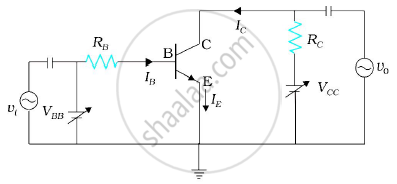Advertisement Remove all ads

# Derive the Expression for the Voltage Gain of a Transistor Amplifier in Ce Configuration in Terms of The Load Resistance Rl, Current Gain a βA and Input Resistance. - Physics

Answer in Brief

Derive the expression for the voltage gain of a transistor amplifier in CE configuration in terms of the  load resistance RL, current gain a βa and input resistance.
Explain why input and output voltages are in opposite phase.

Advertisement Remove all ads

#### Solution

To operate the transistor as an amplifier it is necessary to fix its operating point somewhere in the middle of its active region. If we fix the value of VBB corresponding to a point in the middle of the linear part of the transfer curve then the dc base current Iwould be constant and corresponding collector current I will also be constant. The dc voltage VCE = VCC - ICRwould also remain constant. The operating values of VCE and  IB determine the operating point, of the amplifier.If a small sinusoidal voltage with amplitude vs is superposed on the dc base bias by connecting the source of that signal in series with the VBB supply,  then the base current will have sinusoidal variations  superimposed on the value of IB. As a consequence the collector current also will have sinusoidal  variations superimposed on the value of IC, producing in turn corresponding change in the value of VO.  We can measure the ac variations across the input and output terminals by blocking the dc voltages by large capacitors.

In the discription of the amplifier given above we have not considered any ac signal. In general, amplifiers are used to amplify alternating signals. Now let us superimpose an ac input signal vi (to be amplified) on  the bias VBB (dc) as shown in figure. The output is taken between the collector and the ground.

The working of an amplifier can be easily understood, if we first assume that vi = 0. Then applying  Kirchhoff’s law to the output loop, we get

Vcc = VCE + Ic RL ——— (i)

Likewise, the input loop gives

VBB = VBE + IB RB ——— (ii)

When vi is not zero, we get

VBB + vi = (VBE + ΔVBE) + (IB + ΔIB)R

Or , VBB + vi = VBE + IB RB + ΔVBE + ΔIBRB

The change in VBE can be related to the input resistance ri and the change in IB as Δ VBE = ΔIB ri

So we get, VBB + vi = VBE + IB RB + ΔIB(RB + ri) —— (iii)

Subtracting (ii) from (iii) we get

vi = ΔIB (RB + ri) = r ΔIB ——— (iv)

The change in IB causes a change in Ic. We define a parameter βac, which is similar to the βdc, as

beta_ac = (Delta I_c)/(Delta I_B) = (i_c)/(i_b)   ——— (v)

which is also known as the ac current gain Ai. Usually βac, is close to βdc in the linear region of the output characteristics.

The change in Ic due to a change in IB causes a change in VCE and the voltage drop across the resistor RL because VCC is fixed.

These changes can be given by Eq. (i) as

ΔVCC = ΔVCE + RL ΔIC = 0

or ΔVCE = –RL ΔI

The change in VCE is the output voltage v0. From Eq. (v), we get

v0 = ΔVCE = - βac RL ΔlB  ——— (vi)

The voltage gain of the amplifier is

A_v = (v_0)/(v_i) = (Delta V_(CE))/(rDelta I_B) = (-beta _(ac) R_L)/r       {using eq. (iv) and (vi) }

The negative sign represents that output voltage is opposite in phase with the input voltage.

Is there an error in this question or solution?
Advertisement Remove all ads

#### APPEARS IN

Advertisement Remove all ads
Advertisement Remove all ads
Share
Notifications

View all notifications

Forgot password?